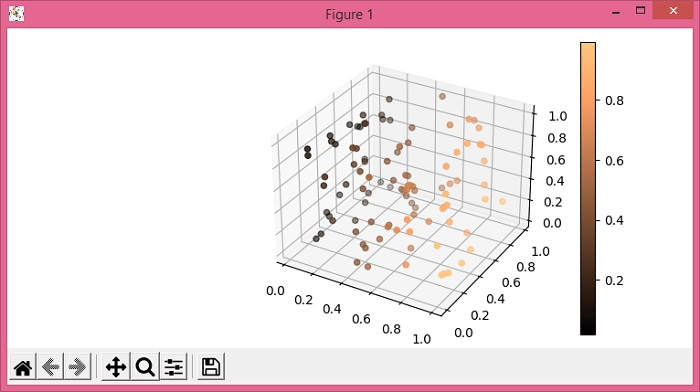# How to plot scatter points in a 3D figure with a colorbar in Matplotlib?

To plot scatter points in a 3D figure with a colorbar in matplotlib, we can use the scatter() and colorbar() methods.

## Steps

• Set the figure size and adjust the padding between and around the subplots.

• Create a new figure or activate an existing figure using figure() method.

• Add an axis as a subplot arrangement.

• Create xs, ys and zs data points using numpy.

• Use scatter() method to create a scatter plot.

• Use colorbar() method with scatter scalar mappable instance for colorbar.

• To display the figure, use show() method.

## Example

import numpy as np
from matplotlib import pyplot as plt
plt.rcParams["figure.figsize"] = [7.50, 3.50]
plt.rcParams["figure.autolayout"] = True
fig = plt.figure()
plt.show()